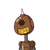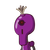# if p and q are the zeros of the quadratic polynomial p(x)= 4x^2-5x-1, find the value of p^2q+pq^2​

if p and q are the zeros of the quadratic polynomial p(x)= 4x^2-5x-1, find the value of p^2q+pq^2​

### 2 thoughts on “if p and q are the zeros of the quadratic polynomial p(x)= 4x^2-5x-1, find the value of p^2q+pq^2​”

1.Step-by-step explanation:

## Given :–

p and q are the zeros of the quadratic polynomial p(x)= 4x^2-5x-1

## To find :–

Find the value of p^2q+pq^2 ?

## Solution:–

Given quardratic polynomial P(x)=4x^2-5x-1

On Comparing this with the standard quadratic Polynomial ax^2+bx+c

We have

a = 4

b = -5

c = -1

Given that

p and q are the zeores of P(x)

We know that

The sum of the zeroes = -b/a

=>p+q = -(-5)/4

=> p+q = 5/4 ——-(1)

and

The product of the zeroes = c/a

=> p×q = -1/4

=> pq = -1/4 ——–(2)

Now

p^2q + pq^2

=> pq(p+q)

From (1)&(2) then

=> (-1/4)(5/4)

=> (-1×5)/(4×4)

=> -5/16

The value of p^2q + pq^2 for the given problem is 5/16

## Used formulae:–

• The standard quadratic Polynomial is ax^2+bx+c.
• Sum of the zeores = -b/a
• The product of the zeroes = c/a
2.